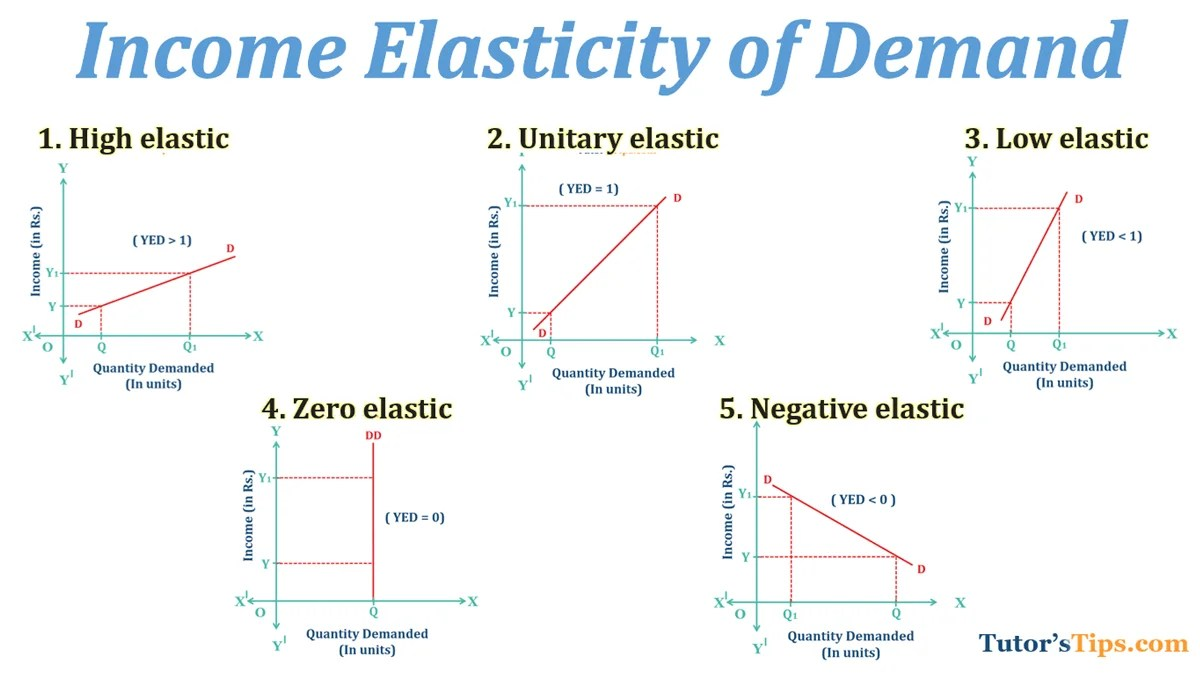# Income elasticity of demand and explained its typesWhen other things remaining constant, the percentage or proportionate change in income results in the percentage or proportionate change in demand of a commodity, it is known as Income Elasticity of Demand.

## Meaning of Income Elasticity of Demand :

It refers to the ratio of the percentage of change in quantity demanded and percentage change in income level of consumer. Therefore, It measures the degree of sensitivity of quantity demanded to change in income.

Hence, It can be calculated as the percentage change in quantity demanded divided by the percentage change in income of the consumer.Here,  % Δ quantity demanded = percentage change in quantity demanded% Δ Income of Consumer = percentage change in Income of Consumer

Thus, it indicates that Higher the income elasticity, more sensitive will be the demand with respect to income. Also, It can be positive or negative depends upon the type of goods demanded whether normal or inferior.

## Definitions:

According to Watson,

“Income Elasticity of demand means the ratio of the percentage change in the quantity demanded to the percentage change in income.”

In the words of Richard G. Lipsey,

“The responsiveness of demand to changes in income is termed as income elasticity of demand.”

For example, suppose a consumer’s income is increased by 10% which results in a rise in demand by 10 %, then income elasticity will be 10%/10% = 1. This implies, the commodity is a normal good.

Similarly, if a 15% hike in the income of consumers declines the demand for commodities by 4.5 %, then income elasticity will be -4.5%/15% = -0.3. It implies the commodity is inferior good.

## Types of Income Elasticity of demand :

1. High elastic
2. Unitary elastic
3. Low elastic
4. Zero elastic
5. Negative elastic

### 1. High Elastic:

The income elasticity of demand can be said as high if the proportionate change in quantity demanded is proportionately more than the increase in income. Therefore, It can be regarded as a positive income elasticity.

For example, suppose the income of Mr A is increased by 20%. As a result, his quantity demanded is increased by 50%. In such a case, the income elasticity is high i.e. YED>1.

### 2. Unitary Elastic:

When the proportionate change in quantity demanded is equal to proportionate change in income, it can be said as unitary income elasticity. For example, suppose the income of a consumer is increased by 50% which leads to rising in quantity demanded by 50%. In such a case, the elasticity would be called unitary i.e. YED=1.

### 3. Low Elastic:

When the proportionate change in quantity demanded is less than the proportionate change in income, it can be regarded as low-income elasticity. For example, let us assume the income of Sumit is increased by 50% but he extended his quantity demanded by 25% only. In such a case, the income elasticity is low i.e. YED<1.

### 4. Zero Elastic:

It can be said as zero when there is no change in quantity demanded with respect to change in income. For example, in the case of necessary goods, the income elasticity is zero as there is no effect of the increase in consumer’s income on his consumption. i.e. YED=0.

### 5. Negative Elastic:

It refers to the situation where an increase in income leads to a fall in quantity demanded. The income elasticity is negative particularly for inferior goods as well as for Giffen goods. For example, if the income of a consumer is increased, he would prefer to purchase wheat instead of millet. In such a case, millet is inferior to wheat and elasticity is negative.i.e. YED <0.